# NCERT Solutions class 7 Maths Chapter-6 Exercise 6.1

NCERT Solutions Class-7 Maths chapter-6 Triangle and its properties Exercise-6.1 is prepared by academic team of pw all the questions of NCERT text book are solved step by step with proper and detail solutions explaining each and every questions . For More and additional questions of CBSE class 7 maths you can go to class 7 maths sections. NCERT class 7 Maths Solutions is the best way to enhanced your mathematics skill. And pw practice worksheet & question bank will help you a lot .

## NCERT Solutions class 7 Maths Chapter-6 Triangle and its properties

### Solutions of Chapter Triangle and its properties Exercise-6.1

Question 1:

In ∆PQR ,D is the mid-point of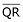.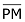is __________.

PD is ___________.

Is QM = MR?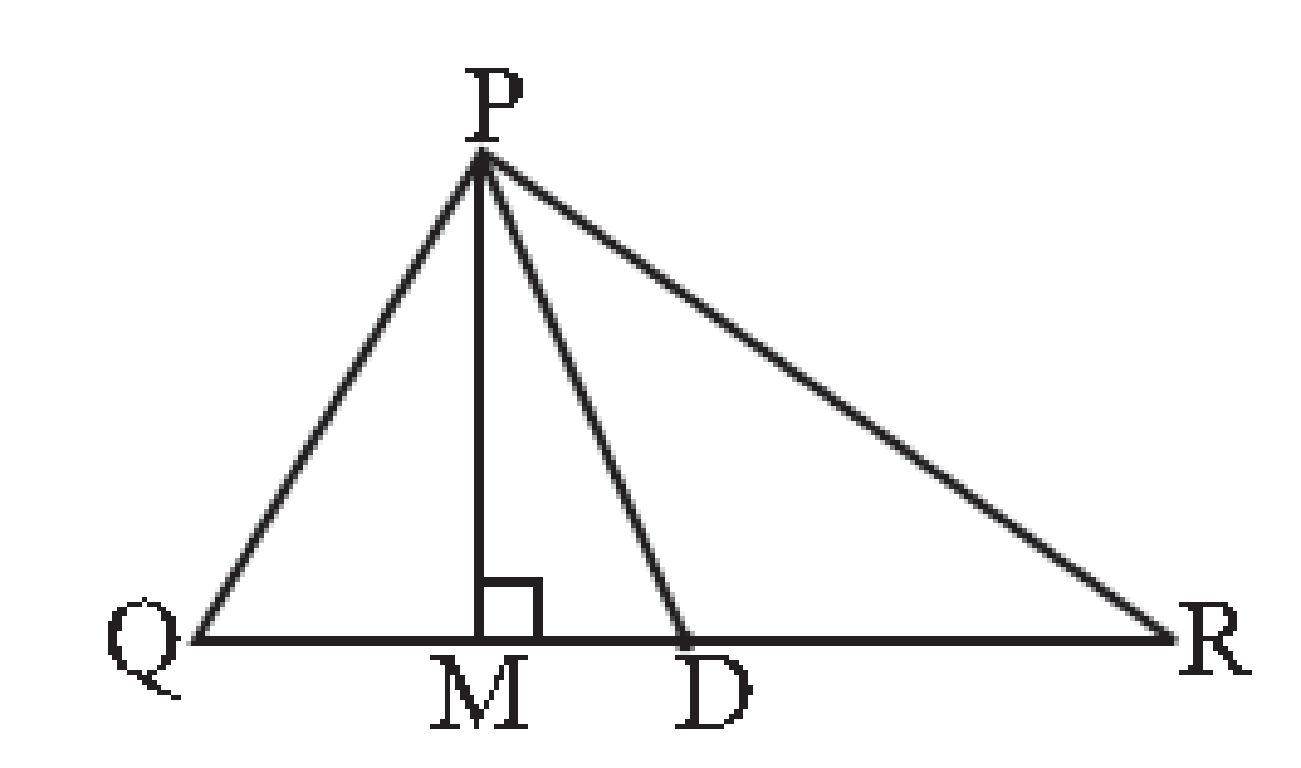In the above given question, we have

∆PQR

Also, given that:

D is the mid-point ofTherefore,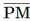is altitude

Also,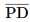is Median

And,

QM ≠ MR

Question 2:

Draw rough sketches for the following:

(a) In ∆ABC, BE is a median.

(b) In  ∆PQR ,PQ and PR are altitudes of the triangle.

(c) In ∆XYZ, YL is an altitude in the exterior of the triangle.

(a) In ∆ABC, BE is a median.

We have,

∆ABC,

And in this triangle BE is a median

Therefore,

The rough sketch for the given figure is as follows: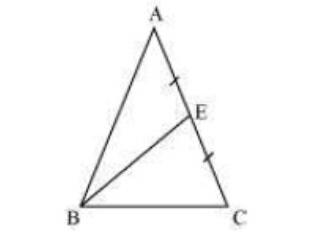(b) In  ∆PQR ,PQ and PR are altitudes of the triangle.

We have,

∆PQR,

And in this triangle PQ and QR are the altitudes of the triangle

Therefore,

The rough sketch for the given figure is as follows: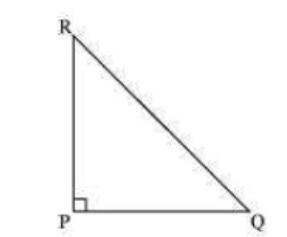(c) In ∆XYZ, YL is an altitude in the exterior of the triangle.

We have,

∆XYZ,

And in this triangle YL is an altitude in the exterior of the triangle

Therefore,

The rough sketch for the given figure is as follows: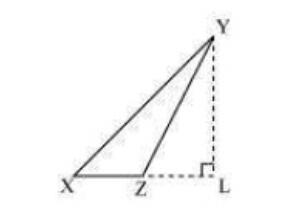Question 3:

Verify by drawing a diagram, if the median and altitude of an isosceles triangle can be same.

We have to prove that:

The median and altitude of an isosceles triangle are same

The diagram for the given question is as follows: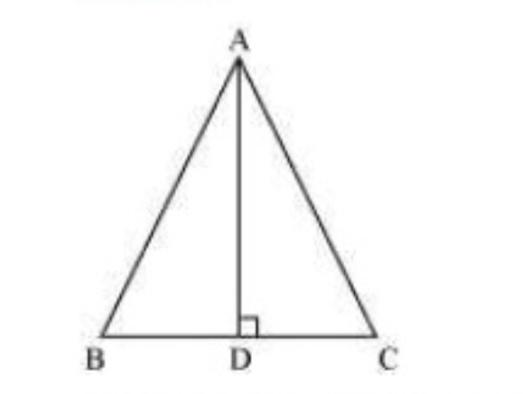This can be proved as follows:

First of all we have to draw a line segment AD which is perpendicular to BC. This is an altitude for this triangle

Now,

From the figure it can also be observed that the length of BD and DC is also same

Therefore,

AD is also median of this isosceles triangle

Hence, proved

NCERT CLASS 7 MATHEMATICS SOLUTIONS